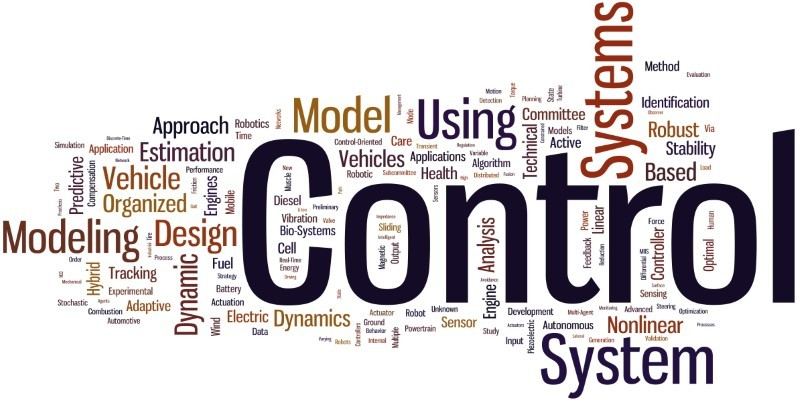# Sparsity Methods for Systems and Control### Sparsity Methods for Systems and Control

The method of sparsity has been attracting a lot of attention in the fields related not only to signal processing, machine learning, and statistics but also systems and control. The method is known as compressed sensing, compressive sampling, sparse representation, or sparse modeling. More recently, the sparsity method has been applied to systems and control to design resource-aware control systems. This book gives a comprehensive guide to sparsity methods for systems and control, from standard sparsity methods infinite-dimensional vector spaces (Part I) to optimal control methods in infinite-dimensional function spaces (Part II).

The primary objective of this book is to show how to use sparsity methods for several engineering problems. For this, the author provides MATLAB programs by which the reader can try sparsity methods for themselves. Readers will obtain a deep understanding of sparsity methods by running these MATLAB programs.

Sparsity Methods for Systems and Control is suitable for graduate-level university courses, though it should also be comprehendible to undergraduate students who have a basic knowledge of linear algebra and elementary calculus. Also, especially part II of the book should appeal to professional researchers and engineers who are interested in applying sparsity methods to systems and control.

•  Introduction (open access (pdf))
• Occam’s Razor
• Group Testing
• Optimization with l1 Norm
• Sparsity Methods for Systems and Control
• What is Sparsity? (slides
• Redundant Dictionary
• Underdetermined Systems
• The l0 Norm
• Exhaustive Search
• Sparse Representation for Functions
• Curve Fitting and Sparse Optimization (slides)
•  Least Squares and Regularization
• Sparse Polynomial and l1-norm Optimization
• Numerical Optimization by CVX
• Algorithms for Convex Optimization(slides)
• Basics of Convex Optimization
• Proximal Operator
• Proximal Splitting Methods for l1 Optimization
• Proximal Gradient Method for l1 Regularization
•  Greedy Algorithms (slides)
•  l0 Optimization
• Orthogonal Matching Pursuit
• Thresholding Algorithm
• Numerical Example
• Applications of Sparse Representation (slides)
• Sparse Representations for Splines
• Discrete-time Hands-off Control
• Dynamical Systems and Optimal Control (slides)
• Dynamical System
• Minimum-time Control
• Minimum-time Control of Rocket
• Maximum Hands-off Control (slides)
• L0 Norm and Sparsity
• Practical Benefits of Sparsity in Control
• Problem Formulation of Maximum Hands-off Control
• L1-optimal Control
• Equivalence Between L0 and L1 Optimal Controls
• Existence of L0-Optimal Control
• Maximum Hands-off Control of Rocket
• Numerical Optimization by Time Discretization (slides)
• Time Discretization
• Controllability of Discretized Systems
• Reduction to Finite-dimensional Optimization
• MATLAB Programs

### What Will You Learn?

• Learn the basics of Sparsity Methods for Systems and Control from A to Z

00:00
00:00
00:00
00:00

### Student Ratings & ReviewsNo Review Yet